# PGPLOT Examples

PGPLOT is designed to make it easy to generate simple graphs, while providing full capability for more complex graphs.

As an example of a simple graph, here is a graph of the function y = x˛ exp(-x), which was drawn with six PGPLOT subroutine calls.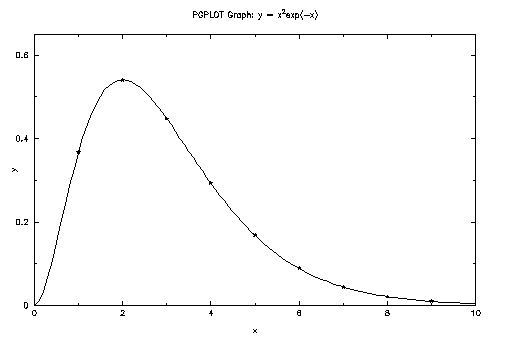This figure is a GIF file. With no change to the source code, the program can also generate files in other formats, such as PostScript.

Here is the Fortran code required to draw this graph, with comments to explain the purpose of each subroutine call. The question mark as an argument to `PGOPEN` allows the user to specify a file name and file format at run time.

 ``` PROGRAM EX1 INTEGER PGOPEN, I REAL XS(9), YS(9), XR(101), YR(101) C Compute numbers to be plotted. DO 10 I=1,101 XR(I) = 0.1*(I-1) YR(I) = XR(I)**2*EXP(-XR(I)) 10 CONTINUE DO 20 I=1,9 XS(I) = I YS(I) = XS(I)**2*EXP(-XS(I)) 20 CONTINUE C Open graphics device. IF (PGOPEN('?') .LT. 1) STOP C Define coordinate range of graph (0 < x < 10, 0 < y < 0.65), C and draw axes. CALL PGENV(0., 10., 0., 0.65, 0, 0) C Label the axes (note use of \u and \d for raising exponent). CALL PGLAB('x', 'y', 'PGPLOT Graph: y = x\u2\dexp(-x)') C Plot the line graph. CALL PGLINE(101, XR, YR) C Plot symbols at selected points. CALL PGPT(9, XS, YS, 18) C Close the graphics device. CALL PGCLOS END ```

The same program can be written in C, using the cpgplot library.

 ```#include "cpgplot.h" #include "math.h" int main() { int i; float xs, ys; float xr, yr; /* Compute numbers to be plotted. */ for (i=0; i<101; i++) { xr[i] = 0.1*i; yr[i] = xr[i]*xr[i]*exp(-xr[i]); } for (i=0; i<9; i++) { xs[i] = i+1; ys[i] = xs[i]*xs[i]*exp(-xs[i]); } /* Open graphics device. */ if (cpgopen("?") < 1) return 1; /* Define coordinate range of graph (0 < x < 10, 0 < y < 0.65), and draw axes. */ cpgenv(0., 10., 0., 0.65, 0, 0); /* Label the axes (note use of \\u and \\d for raising exponent). */ cpglab("x", "y", "PGPLOT Graph: y = x\\u2\\dexp(-x)"); /* Plot the line graph. */ cpgline(101, xr, yr); /* Plot symbols at selected points. */ cpgpt(9, xs, ys, 18); /* Close the graphics device */ cpgclos(); return 0; } ```

More complex graphs supported by PGPLOT include

• Scatter plots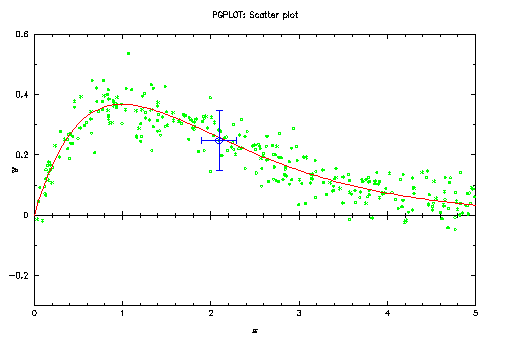• Function plots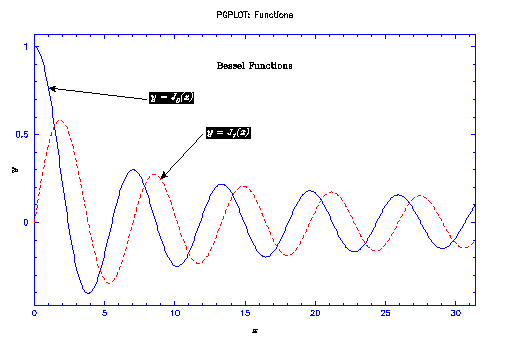• Contour maps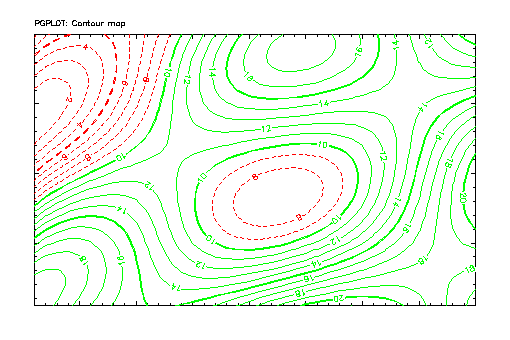• Images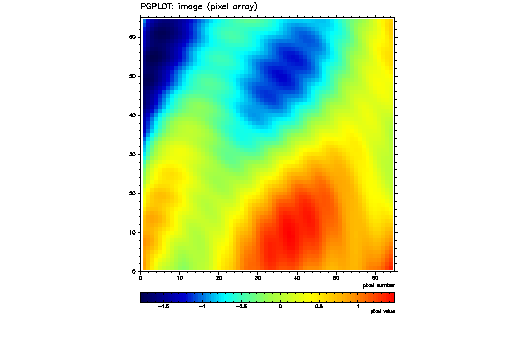• Histograms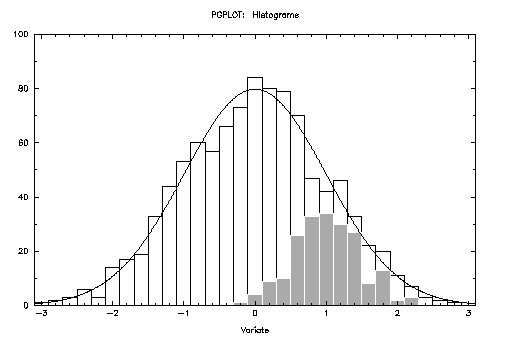All these graphs are also available in a single PostScript file (3 pages).

Further examples can be found in the directory `pgplot/examples` distributed with PGPLOT.

PGPLOT
Tim Pearson, California Institute of Technology, tjp·astro.caltech.edu